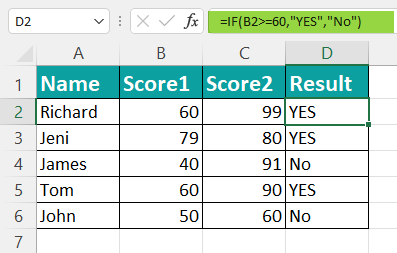# IF Function In ExcelArticle byExcelMojo Team## What is IF Function in Excel?

IF Function in excel tests whether the condition set for the logical text or value is fulfilled or not. If the condition is met, it returns a true value; if not, it returns a false value. In addition to “True” or “False”, the resulting value can be any predefined answer to the logical expression.

For example, the IF Function can be applied as follows:

=IF(A1>B1,”True”,”False”)

Here, if the data in cell A1 is greater than that in the B1 cell, the IF Function will return the “True” value, and if it is vice-versa, the formula will return the “False” value.

### IF() Formula In Excel

• logical_test = It is the logical value or expression for which one seeks a TRUE or FALSE result
• value_if_true = It is the value resulting for the logical_test condition TRUE
• value_if_false = It is another value resulting for the logical_test condition FALSE

### How To Use IF Function In Excel?

Use of IF function in excel involves some operators in their expressions to perform certain tasks, such as:

Let us look at some IF function in excel examples to understand the formula and how it uses different operators.

### IF Function Excel Examples

#### #1 – Conceptual Example

Maria has a list of students from her classroom in column A as follows:

She wants to check if a particular name is present in that column or not using the IF Function. Here’s how she can check the same:

1. Column A has student names, and column B will return the value to the query. The logical test value against the condition is A2 =”John”.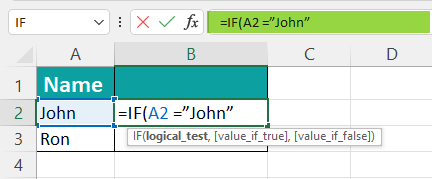2. Start the formula by mentioning the result value as “True” in the formula for cells in the A column containing the name John.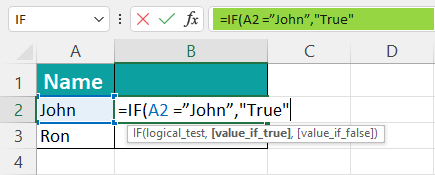3. Mention the result value as “False” in the formula for cells in the A column not containing the name John.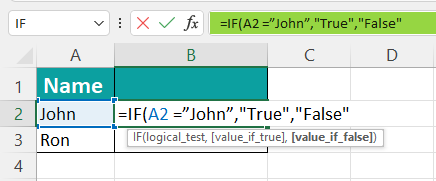4. Finally, execute the IF Function to get the result in column B2.

=IF(A2=”John”,”True”,”False”)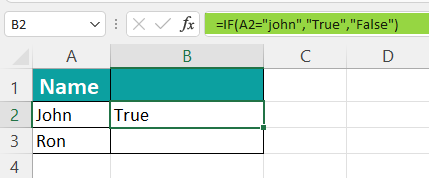5. We will test the condition in all rows of column A. If the A2 cell has “John”, the function will return the value as “True”. Likewise, the A3 cell does not have the name “John”, and hence, the function will return the value as “False”.

6. Drag down the value in the B2 column to where you want it to be.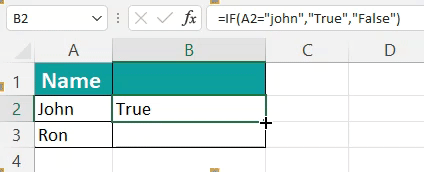#### #2 – IF Function With Text

a. The passing mark for mathematics students in a school exam is 60 out of 100. So, to check which students have passed or failed the exam, we can apply the IF Function in excel.

Column A contains students’ names, while column B contains their marks. Column C will reflect the exam result.

Step 1: The logical test value against the condition is “B2>60”.

Step 2: We can mention the result value as “Pass” in the formula for cells in the B column containing a value greater than 60.

Step 3: We can mention the result value as “Fail” in the formula for cells in the B column containing a value not greater than 60.

Step 4: Finally, we will calculate the IF Function to get the result in column C2.

=IF(B2>60,”Pass”,“Fail”)

Step 5: We can test the condition in all rows of column B. For example, if the B2 cell has a value greater than 60, the function will return the value as “Pass”. Likewise, if the B3 cell does not have a value greater than 60, the function will return the value as “Fail”.

Step 6: If we drag down the value in the C2 column to where we want it to be, the exam result will show up.

b. A fruit vendor named Stephen sells a variety of fruits in his shop. So, he has an inventory checklist in excel to track the stock availability. Column A contains the names of fruits, while column B contains their amount. Finally, column C will show their availability.

He applied the IF Function to learn when to order new fruits. Stephen set the condition that if the stock is less than or equals 20, the value should return as “Order”. But if the stock is not less than or equals 20, the function should return the value as “No Order”.

=IF(B2<=20,”Order”,”No Order”)

c. An art classroom teacher named Lisa needs to assign merit to her students based on their annual scores. So, she prepares an excel sheet with student names and their exam marks. Column A contains the names of students, while column B contains their scores. Column C will calculate their merits.

Lisa executes the IF Function to get the final result. She sets the condition that students with a score more than or equal to 80 will be given Distinction, while those not scoring scores more than or equal to 80 will be Promoted.

=IF(B2>=80,”Distinction”,“Promoted”)

#### #3 Other Applications

a. An IT firm prepares a report to define the tax slab for its employees based on their annual income. Column A contains the names of employees, while column B contains their annual salaries. Finally, column C will calculate if the income is taxable or not.

The company applies the IF Function and sets the condition as salary greater than or equal to ₹250,000 as “Taxable”, while the salary less than or equal to ₹250,000 as non-taxable.

=IF(B2>=250000,”Taxable”,“Non-Taxable”)

b. In another example, a hotel wants to check how much amount should be deducted from the salary of its staff. It prepares excel data, in which column A contains the names of employees while column B contains their annual salary. Column C will highlight the deduction rate.

The hotel uses the IF Function for calculation and sets the condition that the salary less than ₹300,000 will get a 6% deduction, while the salary greater than ₹300,000 will have an 8% deduction.

=IF(B2<300000,”6%”,”8%”)

c. A government organization wants to look at the budget allotted to different departments and decide whether the budget should be passed or not. It creates an excel chart with column A having department names and column B containing their allotted budget. Column C shows the actual funding for departments, while column D will reveal the status of the budget.

It performs the IF Function based on the condition that the allocated budget less than the actual budget will return the value as over budget. In contrast, the allocated budget greater than the actual budget will return the value as acceptable.

=IF (B2<C2,”Over Budget”,”Acceptable”)

### Advanced Examples using IF Function

#### #1 – Nested IF Function In excel

A Nested IF function means using an IF Function within an IF function in excel for multiple conditionsNested IF function in excel tests various criteria, which increases the possibility of outcomes.

For example, there is a group of fruits, and the status of the stock of fruits is calculated.

In column A, fruits are given, the stock is written in column B, and status needs to be calculated in column C.

The IF Function gives output as ‘Order,’ if the amount in stock is less than or equals 20. If the amount in stock is greater than or equals 30, the status shows the result as ‘Sale.’ But if the store has a sufficient number of fruits, the function will return the value as ‘No Order.’

=IF(B2<=20,”Order”,IF(B2>=30,”Sale”,“No Order”))

#### #2 – AND IF Function In Excel

In AND IF Function, both the conditions should be true for the output to be true; else, it will be false.

For example, there is a group of students with their scores in two subjects – score1 and score2. The test condition is if a student has passed or not, i.e., if B2>=60 and C2>=90. If both conditions are true, the output will be true: else, false. So, if Score1 is greater than or equals 60 and Score2 is greater than or equals 90, the result will be “Pass,” else “Fail.”

=IF(AND(B2>=60,C2>=90),”Pass”,“Fail”)

#### #3 – OR IF Function In Excel

In OR IF Function, either condition should be true for the output to be true or false. If the function in excel finds the 1st condition true, it will not check the 2nd condition and give the true output. But if 1st condition is not true, it will check the 2nd one, and if that is true, the output will be true, but if both the conditions are false, then the output will be false.

For example, a group of students is enrolling in swimming classes. The sports teacher checks the students eligible for swimming based on their age and seeks the consent of their parents.

If a student’s age is greater than 7, they are eligible for swimming, and the function will return the value as “Yes”. But if not, the formula will test if the parent permits their child to participate. If yes, they will be eligible for swimming, and the function will return the value as “Yes”. If both conditions are not true, the resulting value will be “No”.

=IF(OR(B2>7, C2=”Yes”),”Yes”,“No”)

### When To Use IF Function?

• IF Function in excel is used in educational institutions to compare students’ marks, merits, etc.
• Companies also use it to compare employees’ performance and salary status.
• Furthermore, it is used in business to compare stocks, prices, performance, etc., of different commodities.
• The IF Function performs operations on the given data easily and efficiently, reducing time and providing the correct result.

### Important Things To Note While Using IF Function In Excel

• There should be two conditions to compare.
• Use the syntax correctly.
• Ensure the cell address, which is to be tested, is accurate.
• The formula must have one test condition (logical expression), which is to be tested and gives output in true or false.
• The test condition is the compulsory argument that should be given and should have logical operators in it.
• True and false values are optional arguments of the IF Function.
• The IF Function can compare a numeric value or string value.
• String value, which should be entered as it is, should be written in double quotes (“”).

1. What is an IF Function in Excel?

IF Function tests logical conditions and gives the output as true or false. In addition, it performs analytical tests for the given data using mathematical operators. The argument can have desired output other than true and false.

For example:

=IF(A1>B1,” True”,” False”)
or,
=IF(A1>B1,” Pass”,” Fail”)

2. How to create IF Function in Excel?

IF Function is created when a logical text or data is tested. It can be any value or string entered in the formula. One can perform some logical conditions on the data through expressions that have logical operators, which provide output according to the test condition. The result can be true or false. If the tested condition is true, the result will be the value of the true argument. But if the tested condition is false, the result will be the value of the false argument.

=IF(logical_test,[value_if_true],[value_if_false])

For Example, the fruit vendor is making the excel sheet for his records. He is testing whether the data is registered correctly or not.

=IF(A1=”Apple”,”Yes”,”No”)

Step 1: The condition is tested as cell A1 has the value “Apple”.
Step 2: If the result is true, the value returns “Yes”.
Step 3: If the result is false, the value returns “No”.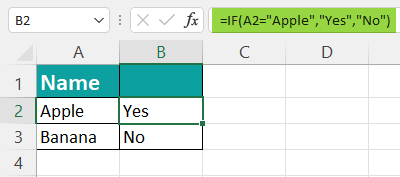3. How to do IF Function in excel with yes or no?

The IF Function is used when any condition is tested. The argument which returns the value “true” or “false” can be replaced with “yes” or “no”.

=IF(logical_test,[value_if_yes],[value_if_no])

For example, a teacher calculates the results of their class. In this table, the scores of the students are mentioned. Score1 of the students are tested that, if it is greater than or equals 60, write the desired output as “yes” or “no”.

=IF(B2>=60,”YES”,”NO”)